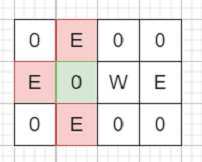# Program to find maximum number enemies will be killed to place a bomb in C++?

Suppose we have a 2D matrix of three different values, 2s, 1s, and 0s, where a 2 represents an enemy, 1 represents a wall and 0 represents an empty cell. We have to find the maximum enemies we can kill using one bomb. The bomb kills all the enemies in the same row and column from the planted point until it hits the wall. And we can put bombs only on blank spaces.

So, if the input is likethen the output will be 3, as we can place the bomb at the green box to kill maximum 3 enemies.

• ret := 0

• n := row count of grid, m := column count of grid

• Define an array colCnt of size m

• for initialize i := 0, when i < n, update (increase i by 1), do:

• for initialize j := 0, when j < m, update (increase j by 1), do:

• if j is zero or grid[i, j] is same as 1, then:

• rowCnt := 0

• if grid[i, j] is same as 1, then:

• k := j + 1

• Otherwise

• k := j

• for k < m and grid[i, k] is not equal to 1, update (increase k by 1), do:

• rowCnt := rowCnt + 1 when (grid[i, k] is 2), otherwise 0

• if i is zero or grid[i, j] is same as 1, then:

• colCnt[j] := 0

• if grid[i, j] is same as 1, then:

• k := i + 1

• Otherwise

• k := i

• for k < n and grid[k, j] is not equal to 1, update (increase k by 1), do:

• colCnt[j] := colCnt[j] + 1 when (grid[k, j] is 2) otherwise 0

• if grid[i, j] is same as 0, then:

• ret := maximum of ret and rowCnt + colCnt[j]

• return ret

Let us see the following implementation to get better understanding:

## Example

Live Demo

#include <bits/stdc++.h>
using namespace std;

class Solution {
public:
int solve(vector<vector<int>>& grid) {
int ret = 0;
int n = grid.size();
int m = n ? grid.size() : 0;
int rowCnt = 0;
vector<int> colCnt(m);
for (int i = 0; i < n; i++) {
for (int j = 0; j < m; j++) {
if (!j || grid[i][j] == 1) {
rowCnt = 0;
int k;
if (grid[i][j] == 1)
k = j + 1;
else
k = j;
for (; k < m && grid[i][k] != 1; k++) {
rowCnt += (grid[i][k] == 2);
}
}
if (!i || grid[i][j] == 1) {
colCnt[j] = 0;
int k;
if (grid[i][j] == 1)
k = i + 1;
else
k = i;
for (; k < n && grid[k][j] != 1; k++) {
colCnt[j] += (grid[k][j] == 2);
}
}
if (grid[i][j] == 0) {
ret = max(ret, rowCnt + colCnt[j]);
}
}
}
return ret;
}
};

main(){
Solution ob;
vector<vector<int>> v = {
{0,2,0,0},
{2,0,1,2},
{0,2,0,0}};
cout << (ob.solve(v));
}

## Input

{{0,2,0,0},
{2,0,1,2},
{0,2,0,0}}

## Output

3